## Introduction

LaTeX is widely used in science and programming has become an important aspect in several areas of science, hence the need for a tool that properly displays code. This article explains how to use the standard verbatim environment as well as the package listings, which provide more advanced code-formatting features. This separate article discusses the minted package, which performs syntax-highlighting using Python's pygmentize library.

## The verbatim environment

The default tool to display code in LaTeX is verbatim, which generates an output in monospaced font.

\begin{verbatim}
Text enclosed inside \texttt{verbatim} environment
is printed directly
and all \LaTeX{} commands are ignored.
\end{verbatim}


The code above produces the following output: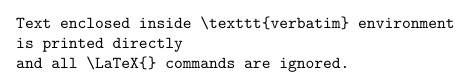Just as in the example at the introduction, all text is printed keeping line breaks and white spaces. There's a starred version of this command whose output is slightly different.

\begin{verbatim*}
Text enclosed inside \texttt{verbatim} environment
is printed directly
and all \LaTeX{} commands are ignored.
\end{verbatim*}


The code above produces the following output: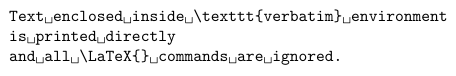In this case spaces are emphasized with a special "visible-space" character: ␣.

Verbatim-like text can also be used in a paragraph by means of the \verb command.

In the directory \verb|C:\Windows\system32| you can find a lot of Windows
system applications.

The \verb+\ldots+ command produces \ldots


The code above produces the following output: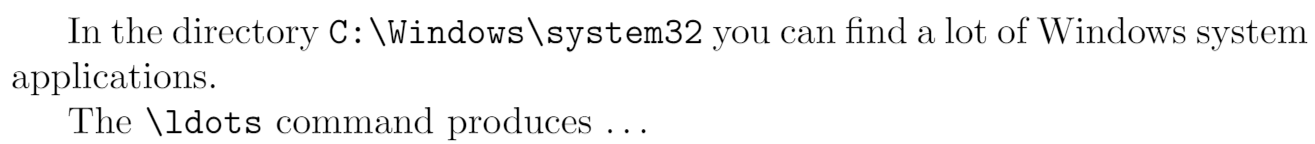The command \verb|C:\Windows\system32| prints the text inside the delimiters | in verbatim format. Any character, except letters and *, can be used as delimiter. For instance \verb+\ldots+ uses + as delimiter.

## Using listings to highlight code

To use the lstlisting environment you have to add the following line to the preamble of your document:

\usepackage{listings}


Here's an example of using the lstlisting environment from the listings package:

\begin{lstlisting}
import numpy as np

def incmatrix(genl1,genl2):
m = len(genl1)
n = len(genl2)
M = None #to become the incidence matrix
VT = np.zeros((n*m,1), int)  #dummy variable

#compute the bitwise xor matrix
M1 = bitxormatrix(genl1)
M2 = np.triu(bitxormatrix(genl2),1)

for i in range(m-1):
for j in range(i+1, m):
[r,c] = np.where(M2 == M1[i,j])
for k in range(len(r)):
VT[(i)*n + r[k]] = 1;
VT[(i)*n + c[k]] = 1;
VT[(j)*n + r[k]] = 1;
VT[(j)*n + c[k]] = 1;

if M is None:
M = np.copy(VT)
else:
M = np.concatenate((M, VT), 1)

VT = np.zeros((n*m,1), int)

return M
\end{lstlisting}


The code above produces the following output: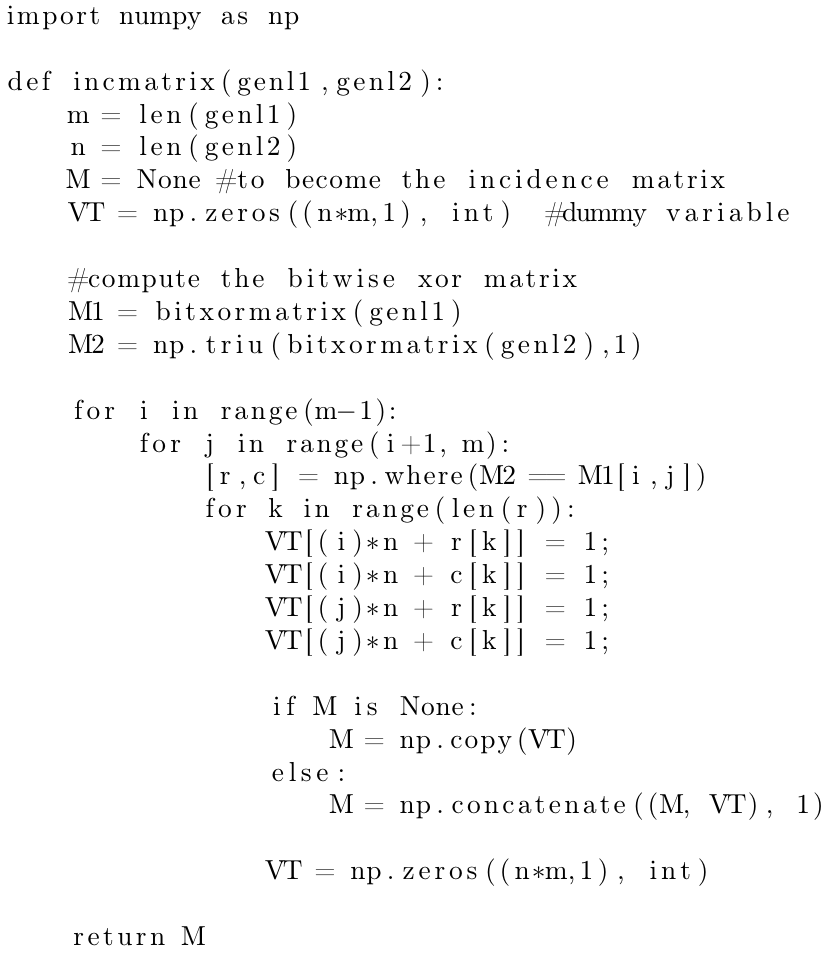In this example, the output ignores all LaTeX commands and the text is printed keeping all the line breaks and white spaces typed. Let's see a second example:

\begin{lstlisting}[language=Python]
import numpy as np

def incmatrix(genl1,genl2):
m = len(genl1)
n = len(genl2)
M = None #to become the incidence matrix
VT = np.zeros((n*m,1), int)  #dummy variable

#compute the bitwise xor matrix
M1 = bitxormatrix(genl1)
M2 = np.triu(bitxormatrix(genl2),1)

for i in range(m-1):
for j in range(i+1, m):
[r,c] = np.where(M2 == M1[i,j])
for k in range(len(r)):
VT[(i)*n + r[k]] = 1;
VT[(i)*n + c[k]] = 1;
VT[(j)*n + r[k]] = 1;
VT[(j)*n + c[k]] = 1;

if M is None:
M = np.copy(VT)
else:
M = np.concatenate((M, VT), 1)

VT = np.zeros((n*m,1), int)

return M
\end{lstlisting}


The code above produces the following output: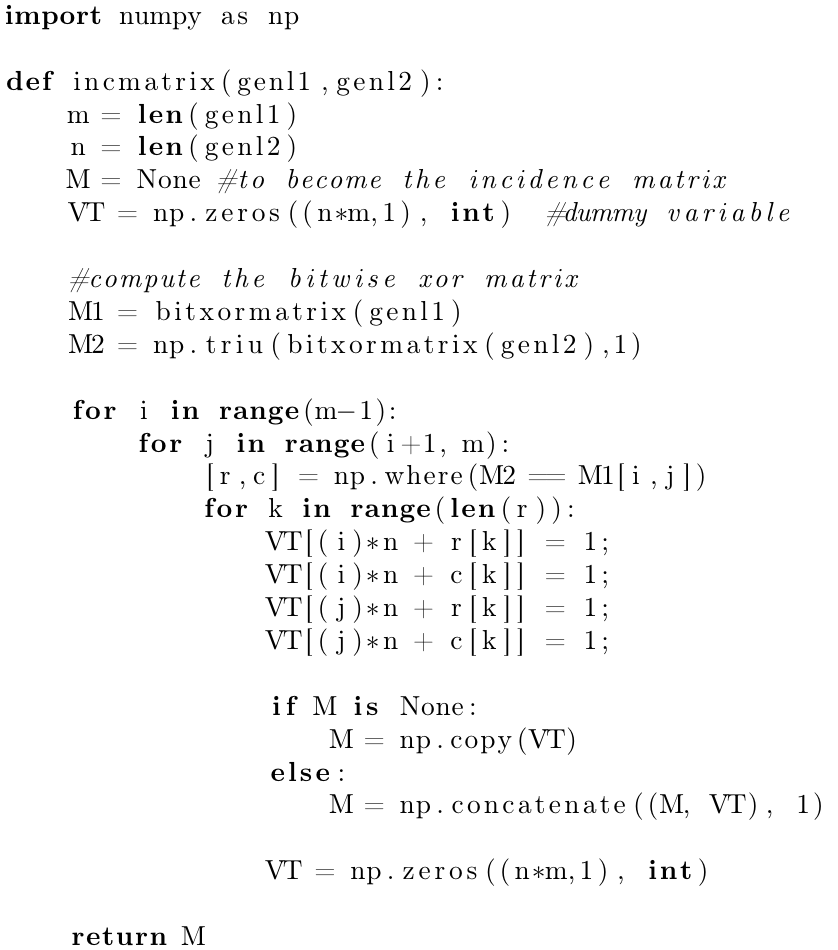The additional parameter inside brackets [language=Python] enables code highlighting for this particular programming language (Python), special words are in boldface font and comments are italicized. See the reference guide for a complete list of supported programming languages.

## Importing code from a file

Code is usually stored in a source file, therefore a command that automatically pulls code from a file becomes very handy.

The next code will be directly imported from a file

\lstinputlisting[language=Octave]{BitXorMatrix.m}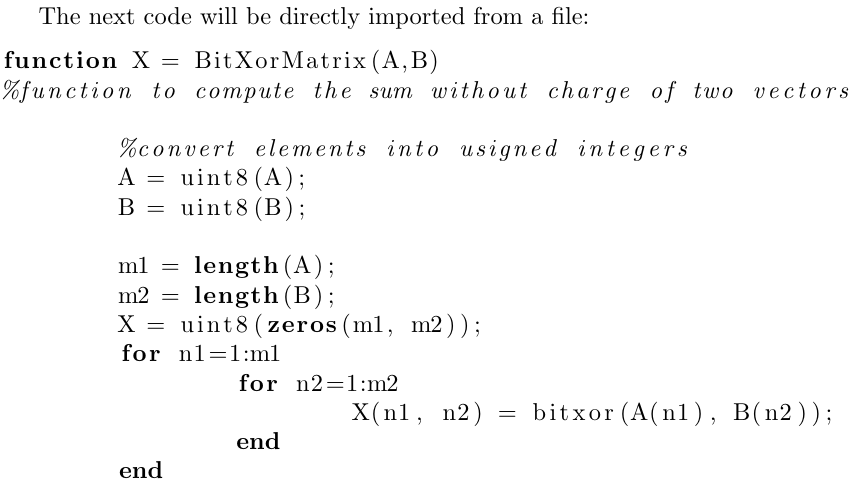The command \lstinputlisting[language=Octave]{BitXorMatrix.m} imports the code from the file BitXorMatrix.m, the additional parameter in between brackets enables language highlighting for the Octave programming language. If you need to import only part of the file you can specify two comma-separated parameters inside the brackets. For instance, to import the code from the line 2 to the line 12, the previous command becomes

\lstinputlisting[language=Octave, firstline=2, lastline=12]{BitXorMatrix.m}


If firstline or lastline is omitted, it's assumed that the values are the beginning of the file, or the bottom of the file, respectively.

## Code styles and colours

Code formatting with the listing package is highly customisable. Let's see an example

\documentclass{article}
\usepackage{listings}
\usepackage{xcolor}

\definecolor{codegreen}{rgb}{0,0.6,0}
\definecolor{codegray}{rgb}{0.5,0.5,0.5}
\definecolor{codepurple}{rgb}{0.58,0,0.82}
\definecolor{backcolour}{rgb}{0.95,0.95,0.92}

\lstdefinestyle{mystyle}{
backgroundcolor=\color{backcolour},
keywordstyle=\color{magenta},
numberstyle=\tiny\color{codegray},
stringstyle=\color{codepurple},
basicstyle=\ttfamily\footnotesize,
breakatwhitespace=false,
breaklines=true,
captionpos=b,
keepspaces=true,
numbers=left,
numbersep=5pt,
showspaces=false,
showstringspaces=false,
showtabs=false,
tabsize=2
}

\lstset{style=mystyle}

\begin{document}
The next code will be directly imported from a file

\lstinputlisting[language=Octave]{BitXorMatrix.m}
\end{document}


The code above produces the following output: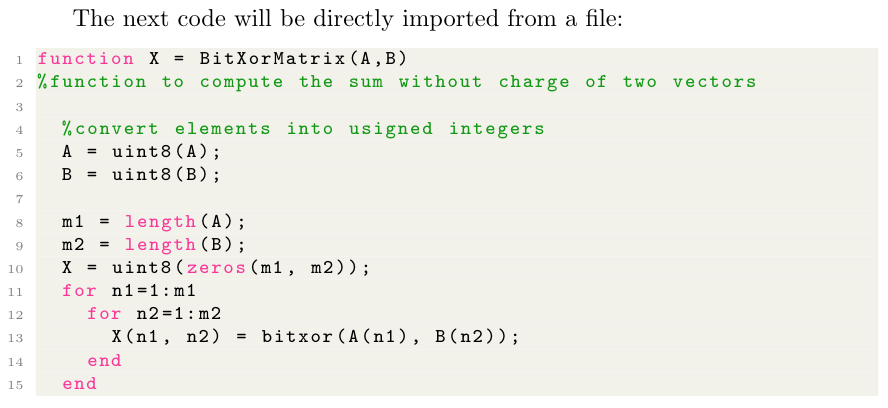As you see, the code colouring and styling greatly improves readability.

In this example the package xcolor is imported and then the command \definecolor{}{}{} is used to define new colours in rgb format that will later be used. For more information see: using colours in LaTeX

There are essentially two commands that generate the style for this example:

\lstdefinestyle{mystyle}{...}
Defines a new code listing style called "mystyle". Inside the second pair of braces the options that define this style are passed; see the reference guide for a full description of these and some other parameters.
\lstset{style=mystyle}
Enables the style "mystyle". This command can be used within your document to switch to a different style if needed.

## Captions and the list of Listings

Just like in floats (tables and figures), captions can be added to a listing for a more clear presentation.

\begin{lstlisting}[language=Python, caption=Python example]
import numpy as np

def incmatrix(genl1,genl2):
m = len(genl1)
n = len(genl2)
M = None #to become the incidence matrix
VT = np.zeros((n*m,1), int)  #dummy variable

#compute the bitwise xor matrix
M1 = bitxormatrix(genl1)
M2 = np.triu(bitxormatrix(genl2),1)

for i in range(m-1):
for j in range(i+1, m):
[r,c] = np.where(M2 == M1[i,j])
for k in range(len(r)):
VT[(i)*n + r[k]] = 1;
VT[(i)*n + c[k]] = 1;
VT[(j)*n + r[k]] = 1;
VT[(j)*n + c[k]] = 1;

if M is None:
M = np.copy(VT)
else:
M = np.concatenate((M, VT), 1)

VT = np.zeros((n*m,1), int)

return M
\end{lstlisting}


The code above produces the following output: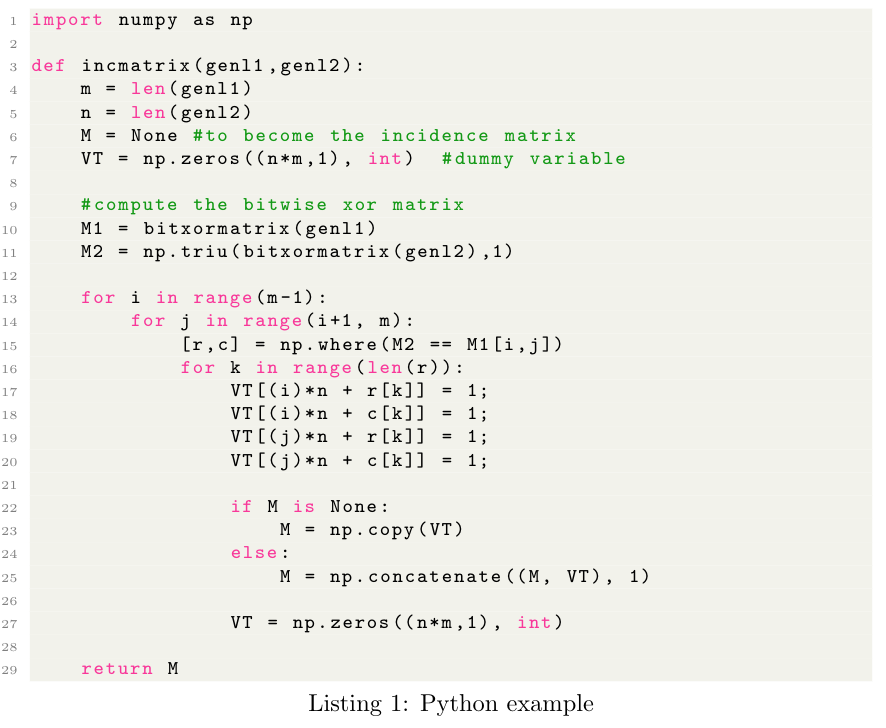Adding the comma-separated parameter caption=Python example inside the brackets, enables the caption. This caption can be later used in the list of Listings.

\lstlistoflistings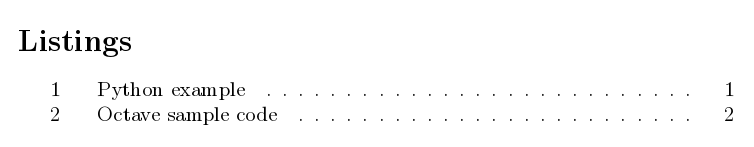## Sample Overleaf project

Open this link to  try out the listings package example on Overleaf.

## Reference guide

### Supported languages

supported languages (and its dialects if possible, dialects are specified in brackets and default dialects are italized):

 ABAP (R/2 4.3, R/2 5.0, R/3 3.1, R/3 4.6C, R/3 6.10) ACSL Ada (2005, 83, 95) Algol (60, 68) Ant Assembler (Motorola68k, x86masm) Awk (gnu, POSIX) bash Basic (Visual) C (ANSI, Handel, Objective, Sharp) C++ (ANSI, GNU, ISO, Visual) Caml (light, Objective) CIL Clean Cobol (1974, 1985, ibm) Comal 80 command.com (WinXP) Comsol csh Delphi Eiffel Elan erlang Euphoria Fortran (77, 90, 95) GCL Gnuplot Haskell HTML IDL (empty, CORBA) inform Java (empty, AspectJ) JVMIS ksh Lingo Lisp (empty, Auto) Logo make (empty, gnu) Mathematica (1.0, 3.0, 5.2) Matlab Mercury MetaPost Miranda Mizar ML Modula-2 MuPAD NASTRAN Oberon-2 OCL (decorative, OMG) Octave Oz Pascal (Borland6, Standard, XSC) Perl PHP PL/I Plasm PostScript POV Prolog Promela PSTricks Python R Reduce Rexx RSL Ruby S (empty, PLUS) SAS Scilab sh SHELXL Simula (67, CII, DEC, IBM) SPARQL SQL tcl (empty, tk) TeX (AlLaTeX, common, LaTeX, plain, primitive) VBScript Verilog VHDL (empty, AMS) VRML (97) XML XSLT

### Options to customize code listing styles

• backgroundcolor - colour for the background. External color or xcolor package needed.
• basicstyle - font size/family/etc. for source (e.g. basicstyle=\ttfamily\small)
• keywordstyle - style of keywords in source language (e.g. keywordstyle=\color{red})
• numberstyle - style used for line-numbers
• numbersep - distance of line-numbers from the code
• stringstyle - style of strings in source language
• showspaces - emphasize spaces in code (true/false)
• showstringspaces - emphasize spaces in strings (true/false)
• showtabs - emphasize tabulators in code (true/false)
• numbers - position of line numbers (left/right/none, i.e. no line numbers)
• prebreak - displaying mark on the end of breaking line (e.g. prebreak=\raisebox{0ex}[0ex][0ex]{\ensuremath{\hookleftarrow}})
• captionpos - position of caption (t/b)
• frame - showing frame outside code (none/leftline/topline/bottomline/lines/single/shadowbox)
• breakatwhitespace - sets if automatic breaks should only happen at whitespaces
• breaklines - automatic line-breaking
• keepspaces - keep spaces in the code, useful for indetation
• tabsize - default tabsize
• escapeinside - specify characters to escape from source code to LaTeX (e.g. escapeinside={\%*}{*)})
• rulecolor - Specify the colour of the frame-box